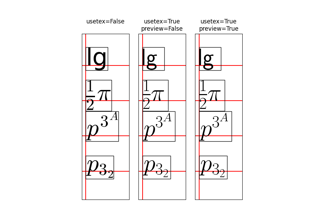matplotlib.axes.subplot_class_factory¶

matplotlib.axes.subplot_class_factory(axes_class=None)[source]

Make a new class that inherits from SubplotBase and the given axes_class (which is assumed to be a subclass of axes.Axes). This is perhaps a little bit roundabout to make a new class on the fly like this, but it means that a new Subplot class does not have to be created for every type of Axes.

Examples using matplotlib.axes.subplot_class_factory¶